The perfect place for easy learning...

×

# Python Lists

In Python, a list is a collection of ordered and indexed elements of different data types. In Python, the list and its elements are mutable. That means, the list and its elements can be modified at any time in the program. In Python, the list data type (data structure) has implemented with a class known as a list. All the elements of a list must be enclosed in square brackets, and each element must be separated with a comma symbol. In Python, the list elements are organized as an array of elements of different data types. All the elements of a list are ordered and they are indexed. Here, the index starts from '0' (zero) and ends with 'number of elements - 1'.

• In Python, the list elements are also indexed with negative numbers from the last element to the first element in the list. Here, the negative index begins with -1 at the last element and decreased by one for each element from the last element to the first element.

## Creating a list in Python

The general syntax for creating a list is as follows.

### Syntax

`list_name = [element_1, element_2, element_3, ...]`

For example, consider the following code for creating a list which stores the details of a student.

# Python code to illustrate creating a list

``````
student_data = [1, 'Rama', '2nd Year', 'CSE', 85.80]
print(student_data)
```
```

When we run the above code, it produces the output as follows.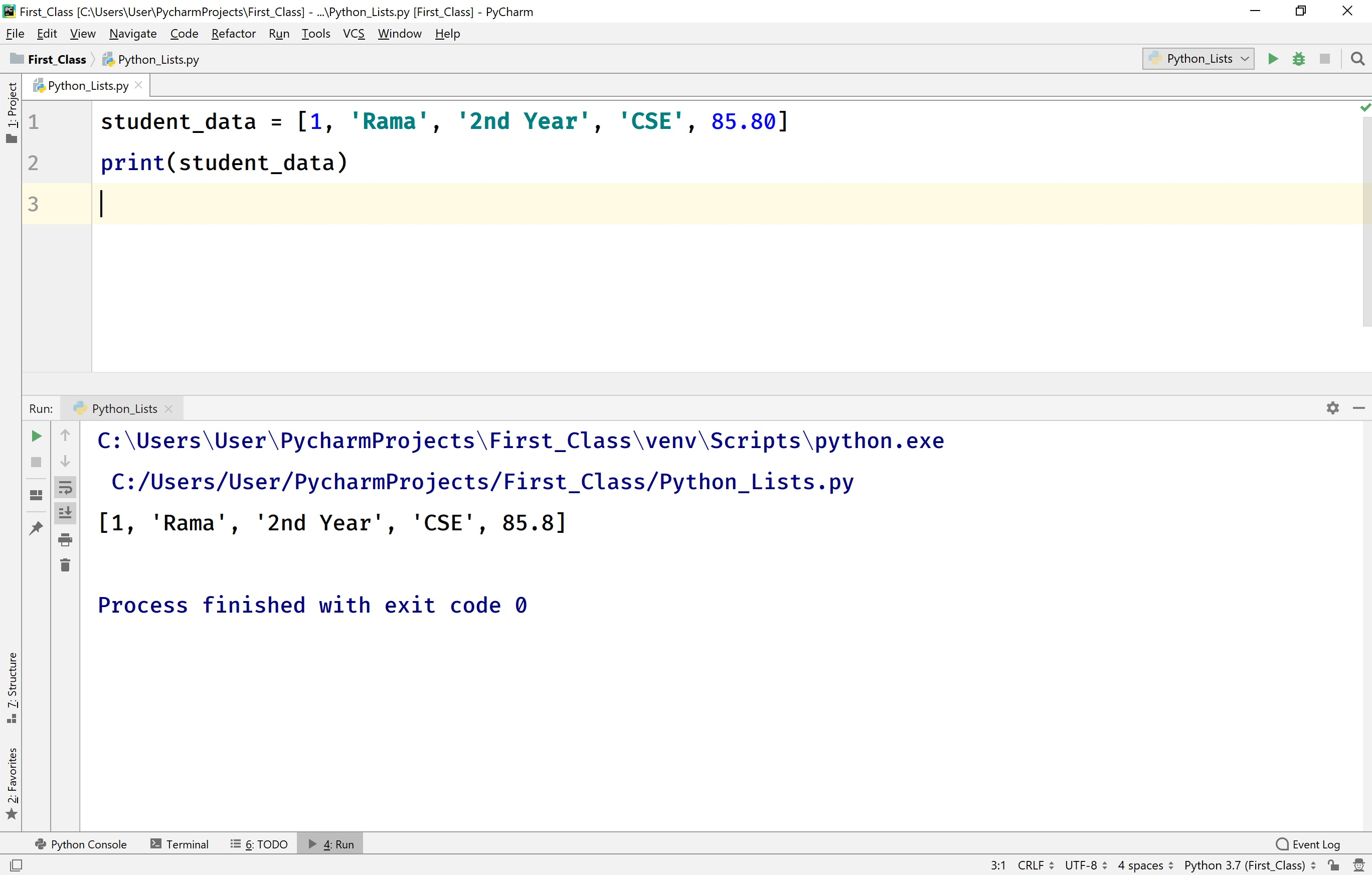In Python, a list can also be created using list() constructor. The list() constructor takes only one argument.

### Syntax

`list_name = list([element_1, element_2, element_3, ...])`

For example, consider the following code for creating a list using list() constructor which stores the details of a student.

# Python code to illustrate creating a list using list() constructor.

``````
student_data = list([1, 'Rama', '2nd Year', 'CSE', 85.80])
print(type(student_data))
print(student_data)
```
```

When we run the above code, it produces the output as follows.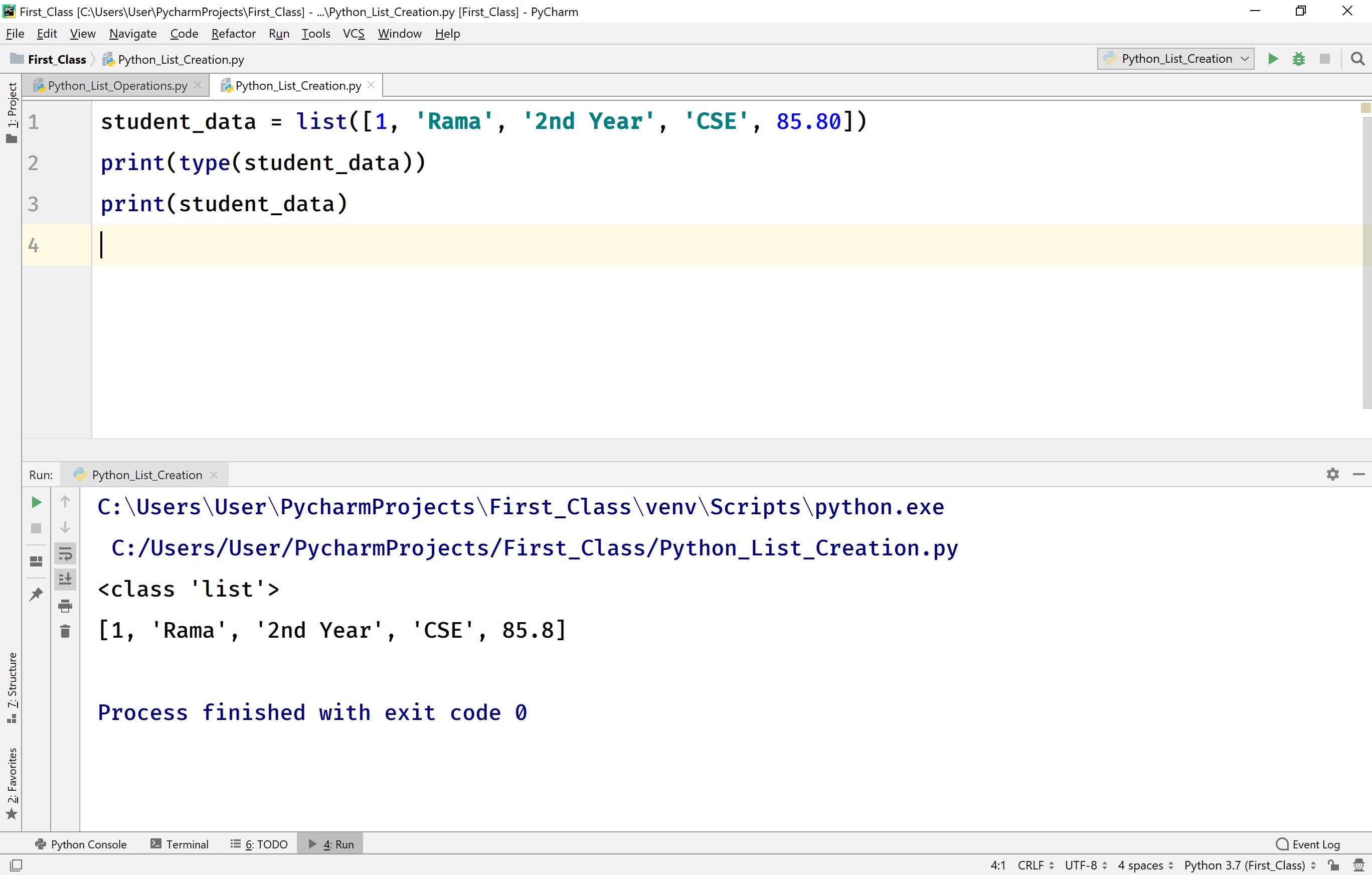## Accessing Elements of a list in Python

In Python, the list elements are organized using index values that start with '0' (zero) at first element and ends with 'length of the list - 1' at last element. The individual elements of a list are accessed using the index values.

### Syntax

`list_name[index]`

For example, consider the following code for accessing individual elements of a list.

# Python code to illustrate accessing elements of a list.

``````
student_data = [1, 'Rama', '2nd Year', 'CSE', 85.80]
print(f'Roll Number: {student_data}\n'
f'Name of the Student: {student_data}\n'
f'Branch: {student_data}\n'
f'Year: {student_data}\n'
f'Percentage: {student_data}')
```
```

When we run the above code, it produces the output as follows.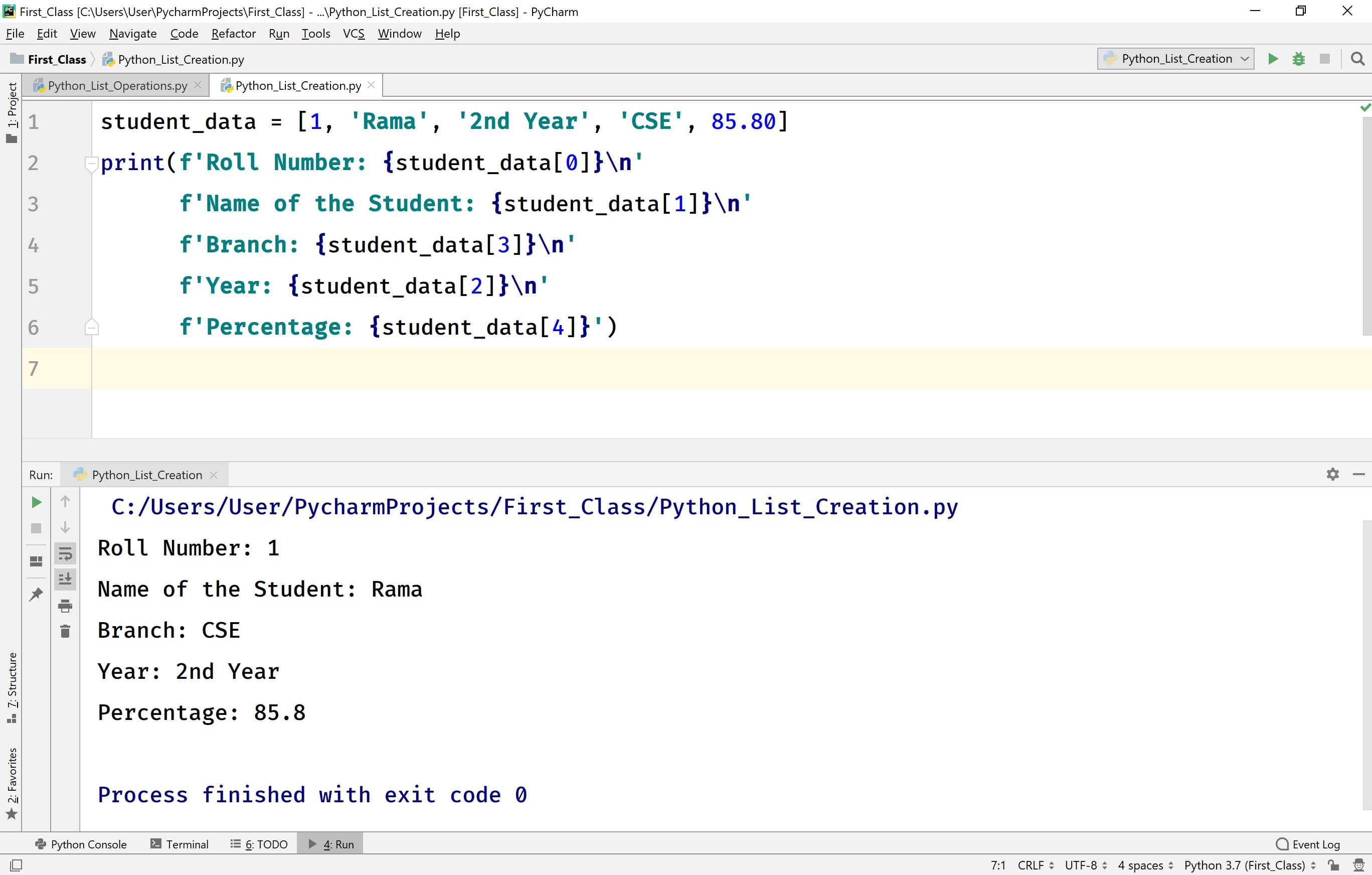In Python, we can also access a subset of elements from a list using slicing. We can access any subset of elements from a specified starting index to ending index. In list slicing, the default starting index is '0' and the default ending is 'length of the list - 1'.

### Syntax

`list_name[starting_index : ending_index]`

For example, consider the following code for accessing a subset of elements from a list.

# Python code to illustrate accessing a subset of elements from a list.

``````
student_data = [1, 'Rama', '2nd Year', 'CSE', 85.80]
print(student_data[2:4]) # Accessing elements from index 2 to 3
print(student_data[:4]) # Accessing elements from index 0 to 3
print(student_data[2:]) # Accessing elements from index 2 to last element
```
```

When we run the above code, it produces the output as follows.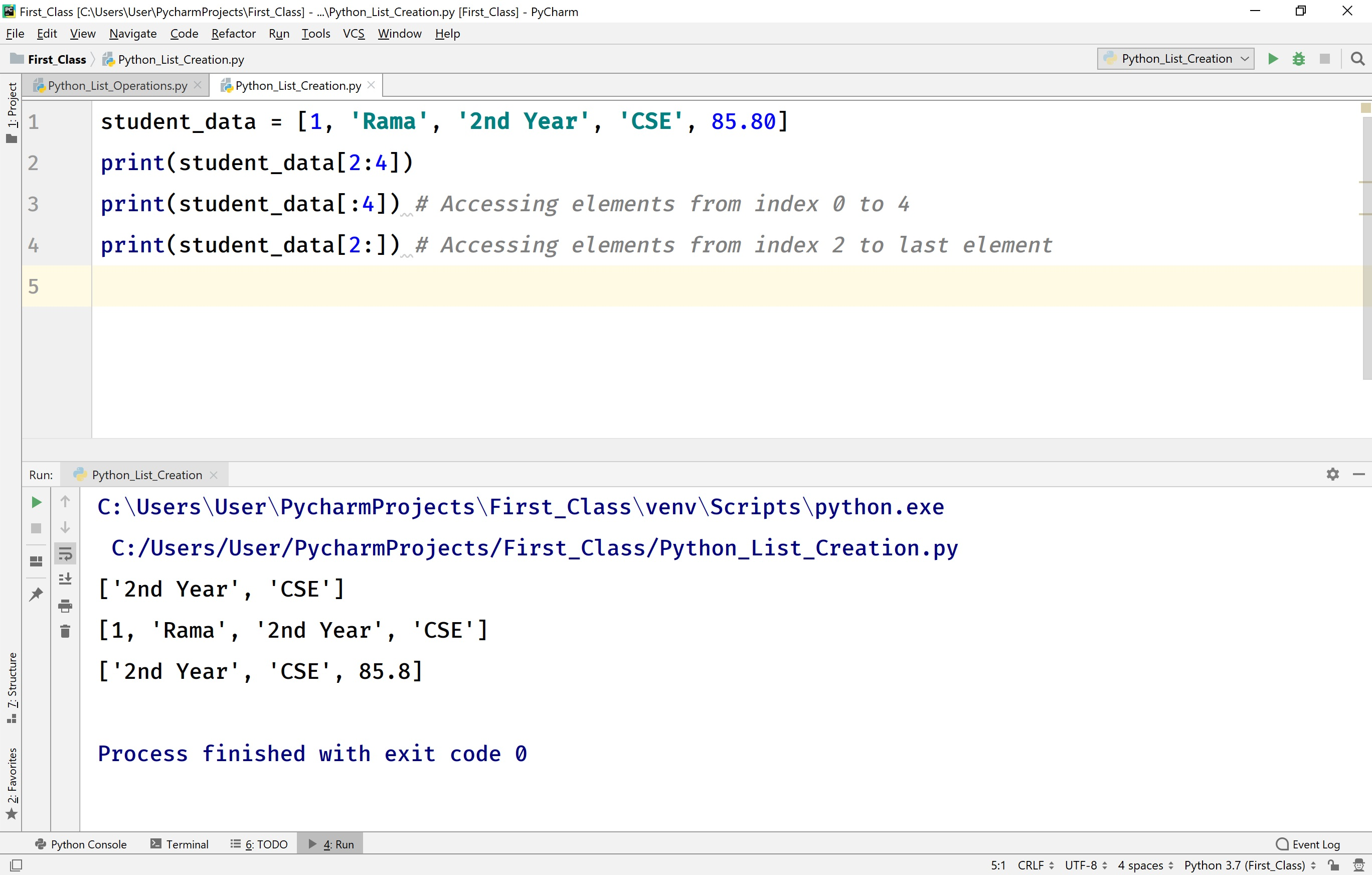## Changing an Element of a list in Python

In Python, an element of a list can be changed at any time using index value.

### Syntax

`list_name[index] = new_value`

For example, consider the following code for changing an element of a list.

# Python code to illustrate changing an element of a list.

``````
student_data = [1, 'Rama', '2nd Year', 'CSE', 85.80]
student_data = 'Seetha'
print(student_data)
```
```

When we run the above code, it produces the output as follows.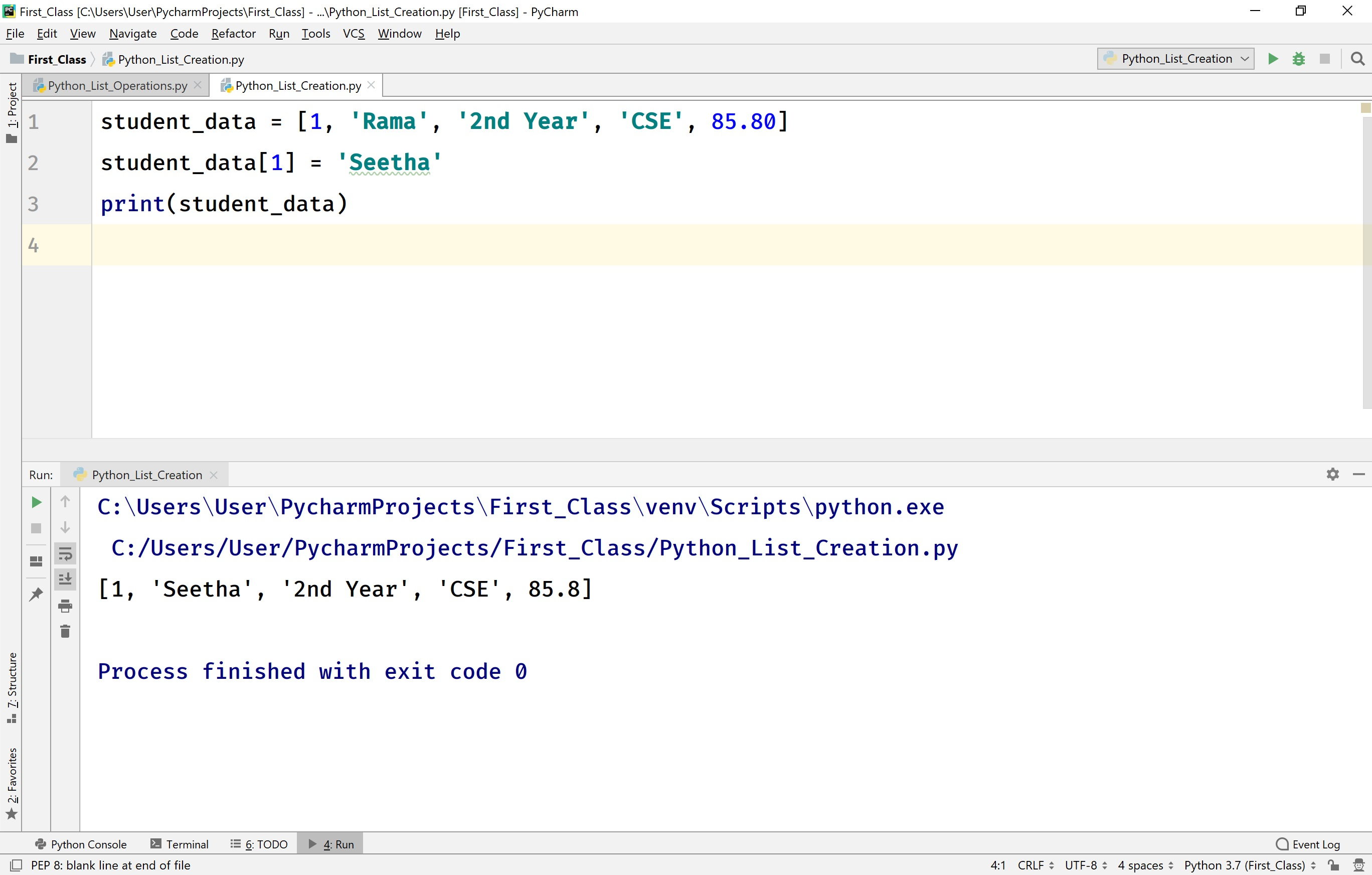## Looping through a list in Python

In Python, we can loop through a list using for statement with membership operator in.

For example, consider the following code to loop through a list.

# Python code to illustrate loop through a list.

``````
student_data = [1, 'Rama', '2nd Year', 'CSE', 85.80]
for element in student_data:
print(f'Element from the List is - {element}')
```
```

When we run the above code, it produces the output as follows.## Existence of an element in a list in Python

In Python, we can test whether an element is present in a list or not using membership operator 'in'.

For example, consider the following code to test the existence of an element in a list.

# Python code to illustrate the existence of an element in a list.

``````
student_data = [1, 'Rama', '2nd Year', 'CSE', 85.80]
if 'CSE' in student_data:
print(f'CSE is found in the list!!!')
else:
```
```

When we run the above code, it produces the output as follows.## Finding the length of a list in Python

The Python provides a built-in function len( ) to find the length of a list. Here, the length of a list is the total number of elements in that list.

For example, consider the following code to find the length of a list.

# Python code to illustrate the length of a list.

``````
student_data = [1, 'Rama', '2nd Year', 'CSE', 85.80]
list_length = len(student_data)
print(f'Length of the list is {list_length}')
```
```

When we run the above code, it produces the output as follows.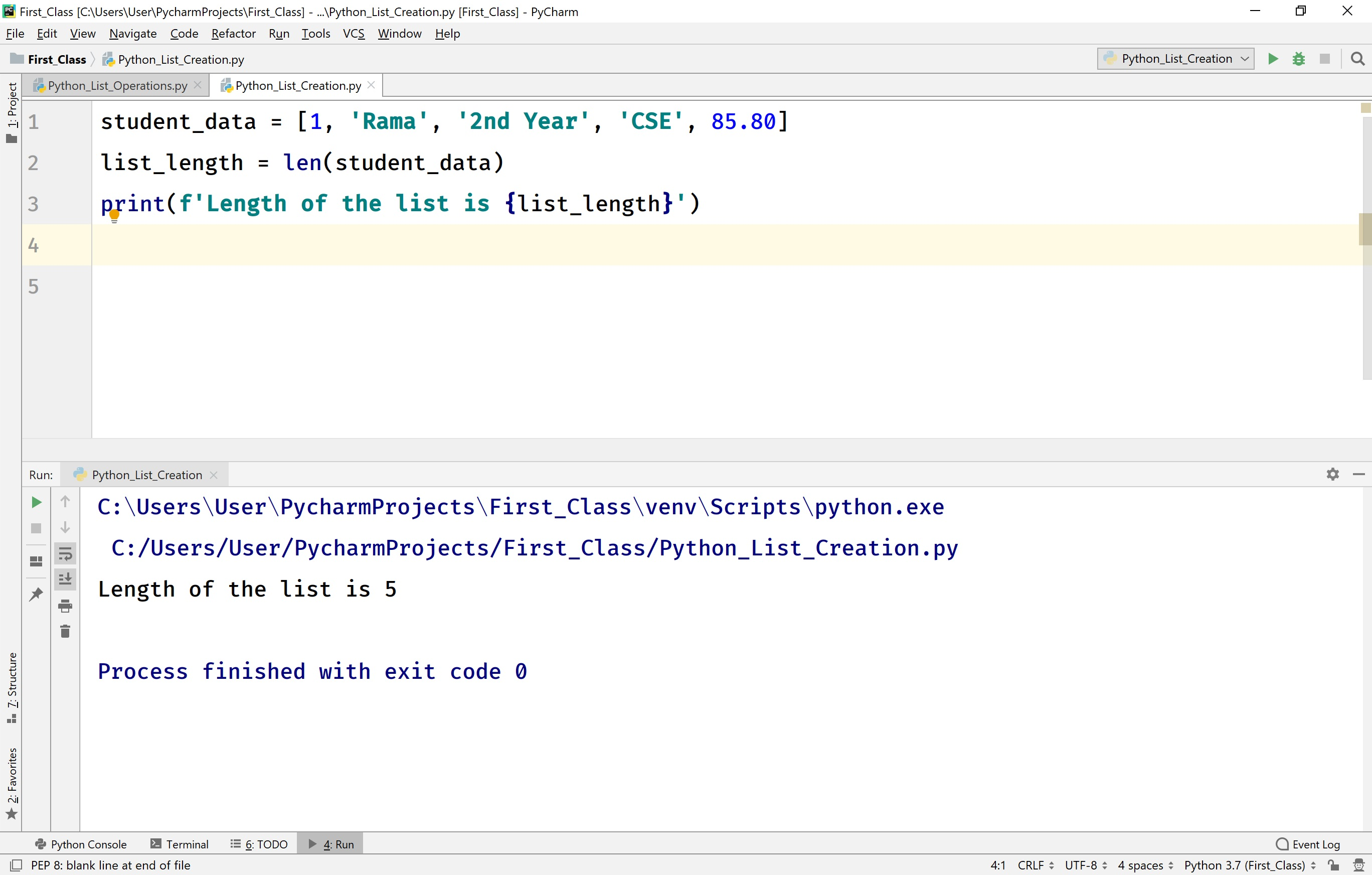## Adding an element to the list in Python

Adding an element to the existing list can be performed using the following built-in methods.

• append(value)
• insert(index, value)

append(value) - This method adds a new element at the end of the list.

For example, consider the following code.

# Python code to illustrate append() method in a list.

``````
student_data = [1, 'Rama', '2nd Year', 'CSE', 85.80]
student_data.append('promoted')
print(f'The list after append is\n{student_data}')
```
```

When we run the above code, it produces the output as follows.insert(index, value) - This method inserts a new element at the specified index of the list.

For example, consider the following code.

# Python code to illustrate insert() method in a list.

``````
student_data = [1, 'Rama', '2nd Year', 'CSE', 85.80]
student_data.insert(2, 'promoted')
print(f'The list after insertion at index 2 is\n{student_data}')
```
```

When we run the above code, it produces the output as follows.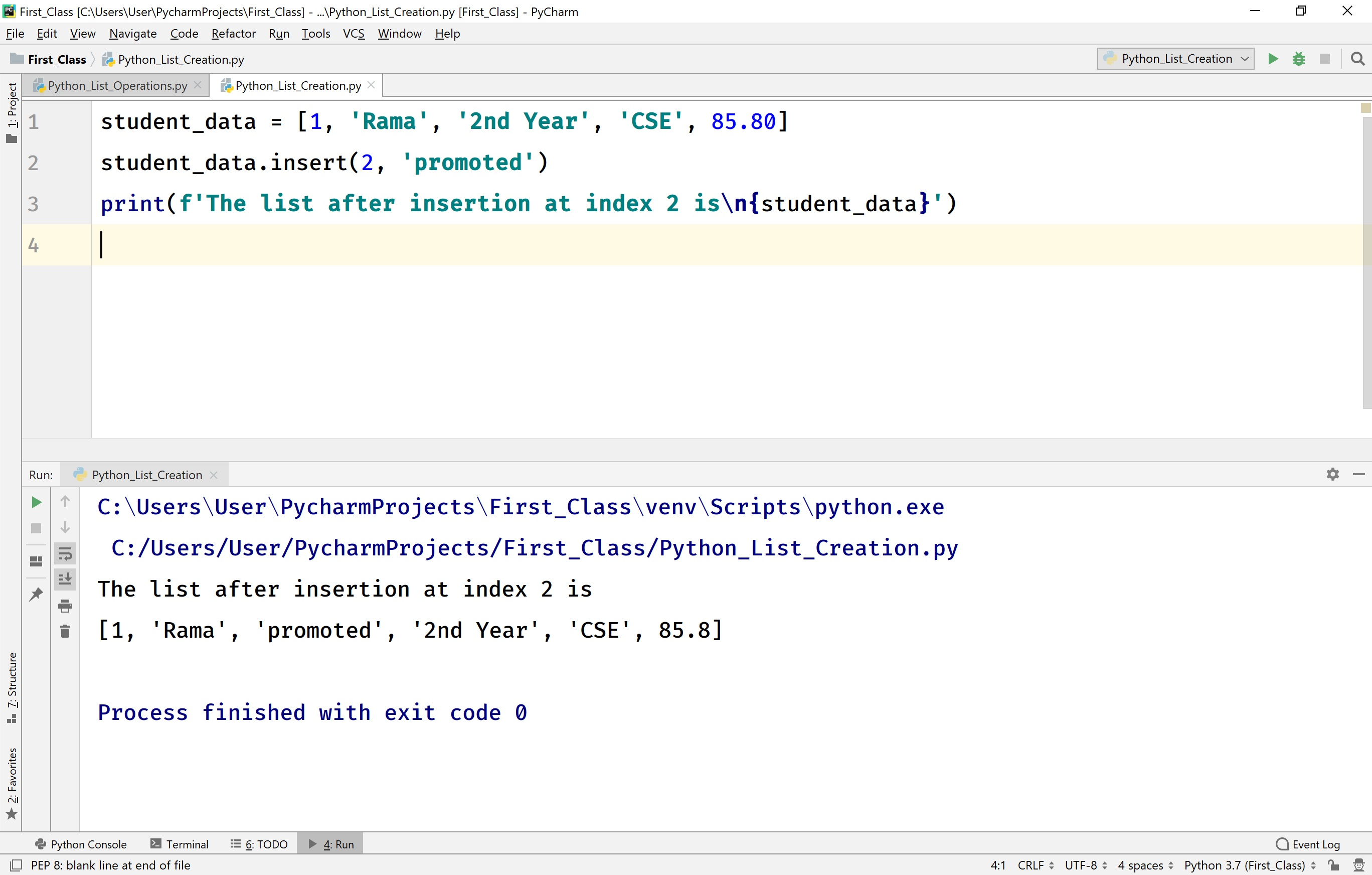• When we use insert( ) method with an index value out of range then, the new element is inserted at the end of the list.

## Removing elements from a list in Python

The Python provides the following built-in methods to remove elements from a list.

• remove(value)
• pop()
• clear()
• del

remove(value) - This method removes the specified element from the list. If the specified element is not found in the list, then the execution terminates with an error message.

pop( ) or pop(index) - This method removes the last element from the list. But, when it is used with an index value, it removes the value at the specified index from the list.

clear( ) - This method removes all the elements from the list. The clear( ) method makes the list empty.

del Keyword - This keyword removes the complete list from the memory. After del keyword used with a list, we can not use it again.

For example, consider the following code.

# Python code to illustrate remove operation in a list.

``````
my_list = [1, 2, 3, 4, 5, 6, 7, 8, 9]
print(f'The list is - {my_list}')
my_list.remove(6)
print(f'The list after removing 6 is - {my_list}')
my_list.pop()
print(f'The list after pop is - {my_list}')
my_list.pop(2)
print(f'The list after pop with index 2 is - {my_list}')
my_list.clear()
print(f'The list after clear is - {my_list}')
del my_list
#print(f'The list after del keyword used is - {my_list}') # GENERATES ERROR
```
```

When we run the above code, it produces the output as follows.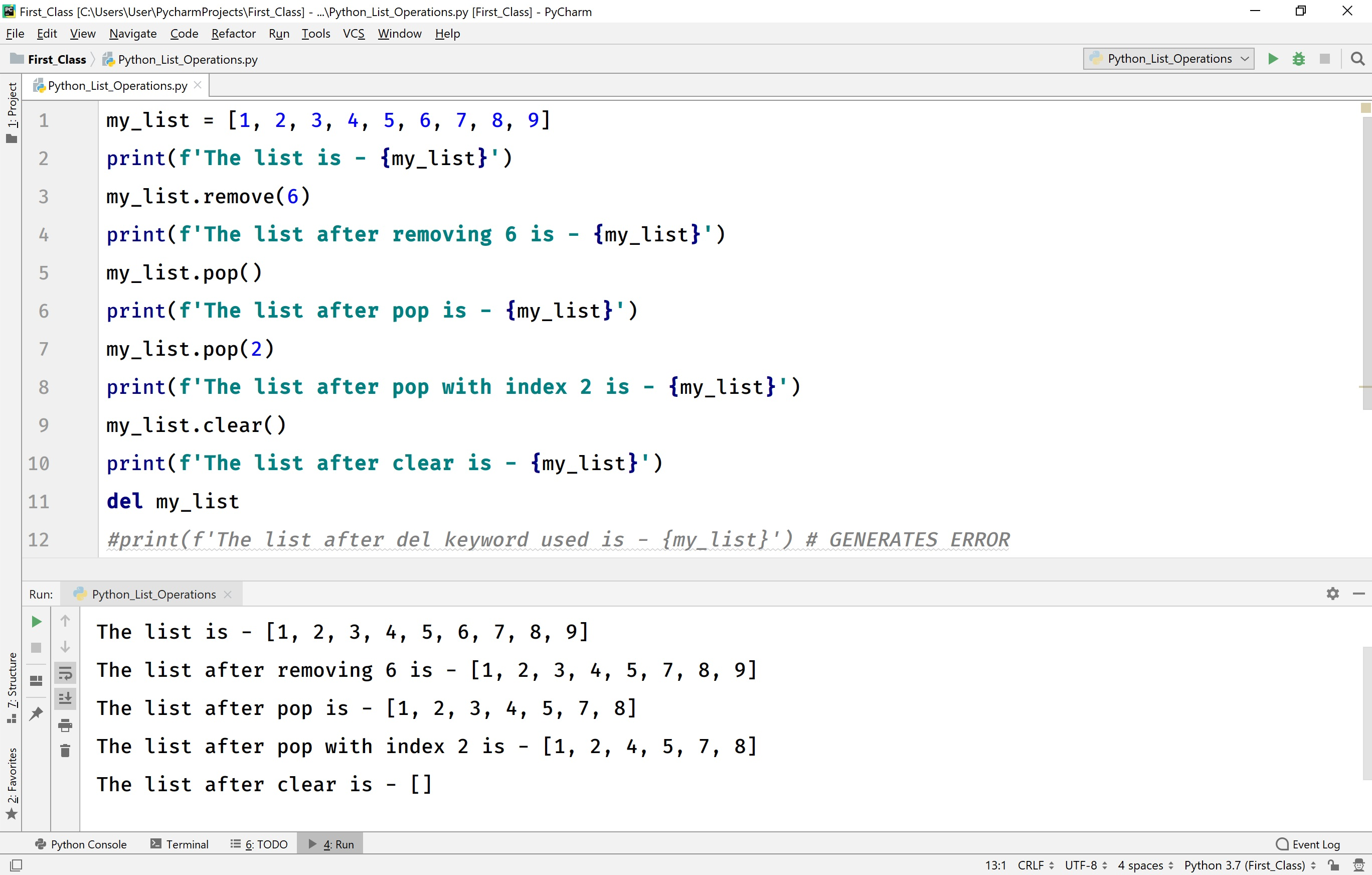## Counting the number of time a value appears in the list

The Python provides a built-in method called count(value) to count the number of times the given value appears in the list.

For example, consider the following code.

# Python code to illustrate the count( ) method in a list.

``````
my_list = [1, 2, 3, 4, 5, 6, 7, 8, 9, 2, 5, 2]
print(f'The list is - {my_list}')
print(f'The value 2 appears {my_list.count(2)} times in the list.')
```
```

When we run the above code, it produces the output as follows.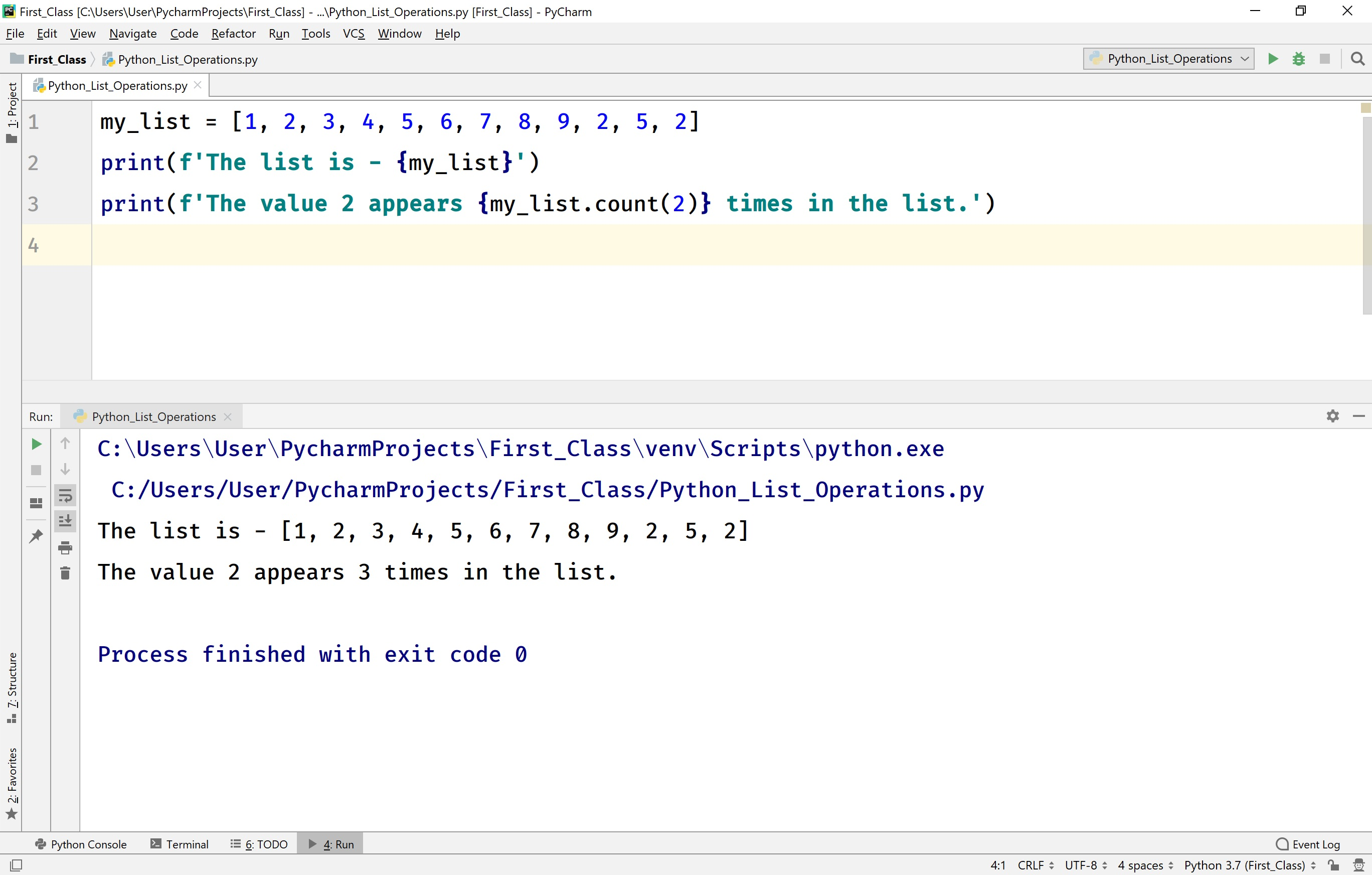## Extending a list

The Python provides a built-in method called extend( ) to append elements of a list to another list. For example, consider the following example.

# Python code to illustrate extend( ) method in a list.

``````
my_list_1 = [1, 2, 3, 4, 5]
my_list_2 = [10, 20, 30]
my_list_1.extend(my_list_2)
print(f'The list_1 after extend is - {my_list_1}')
```
```

When we run the above code, all the elements of my_list_2 are appended to my_list_2. So, it produces the output as follows.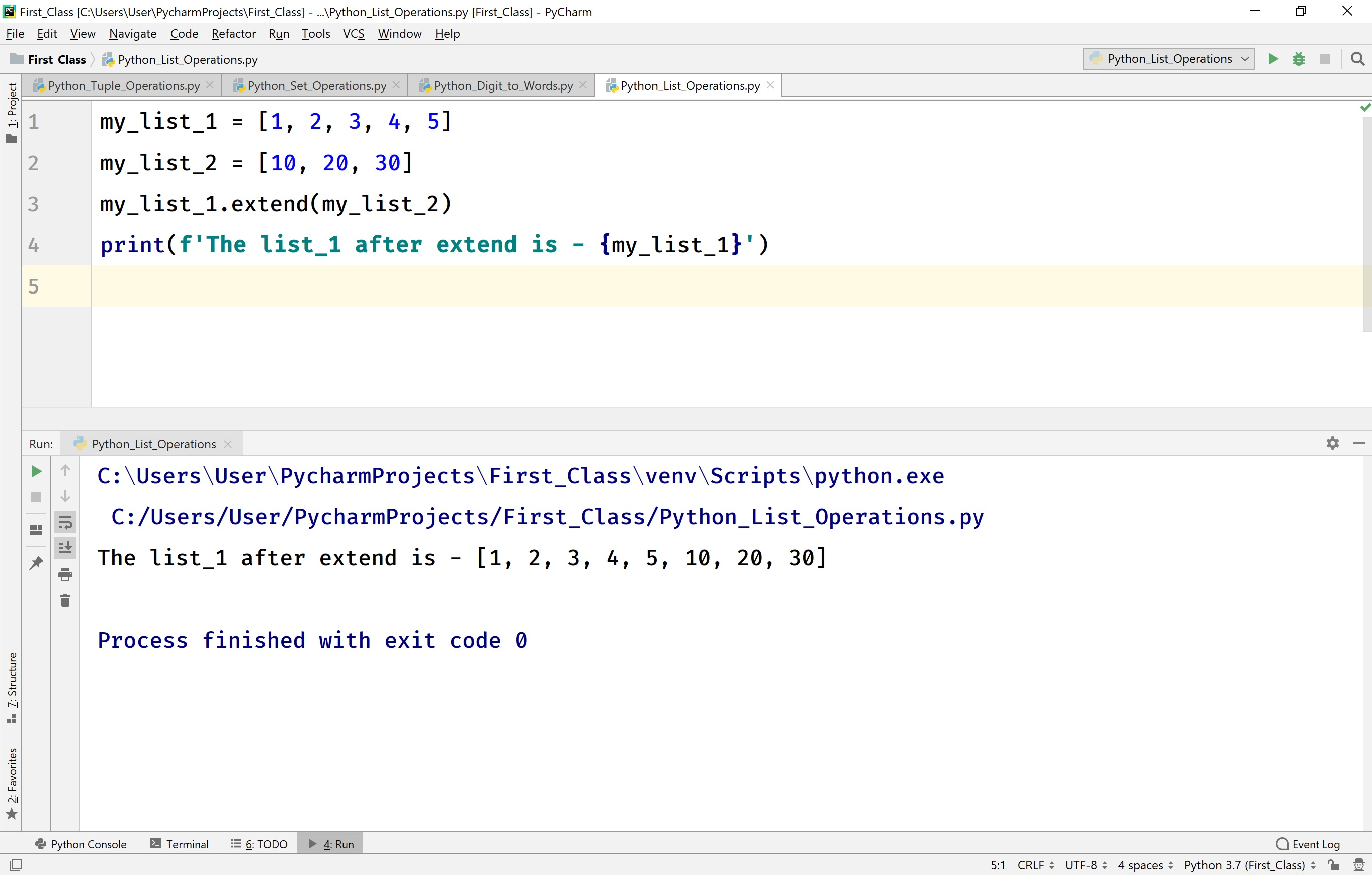## Finding the index of a value in a list

The Python provides a built-in method called index( value ) to find the index of that value in the list. If the given value not found in the list, then the execution terminates with an error message ValueError: <<value>> is not in the list. For example, consider the following example.

# Python code to illustrate the index( ) method in a list.

``````
my_list = [1, 2, 3, 4, 5]
print(f'The index of the value 4 is {my_list.index(4)}')
#print(f'The index of the value 7 is {my_list.index(7)}') # generates an error
```
```

When we run the above code, it produces the output as follows.## Finding Maximum and Minimum value in a list

The Python programming language provides built-in methods max( ) and min( ) to find the maximum and minimum elements in a list.

For example, consider the following code.

# Python code to illustrate max( ) and mim( ) methods in a list.

``````
my_list = [12, 2, 5, 90, 30, 40, 3]
print(f'The maximum value in the list is - {max(my_list)}')
print(f'The minimum value in the list is - {min(my_list)}')
```
```

When we run the above code, it produces the output as follows.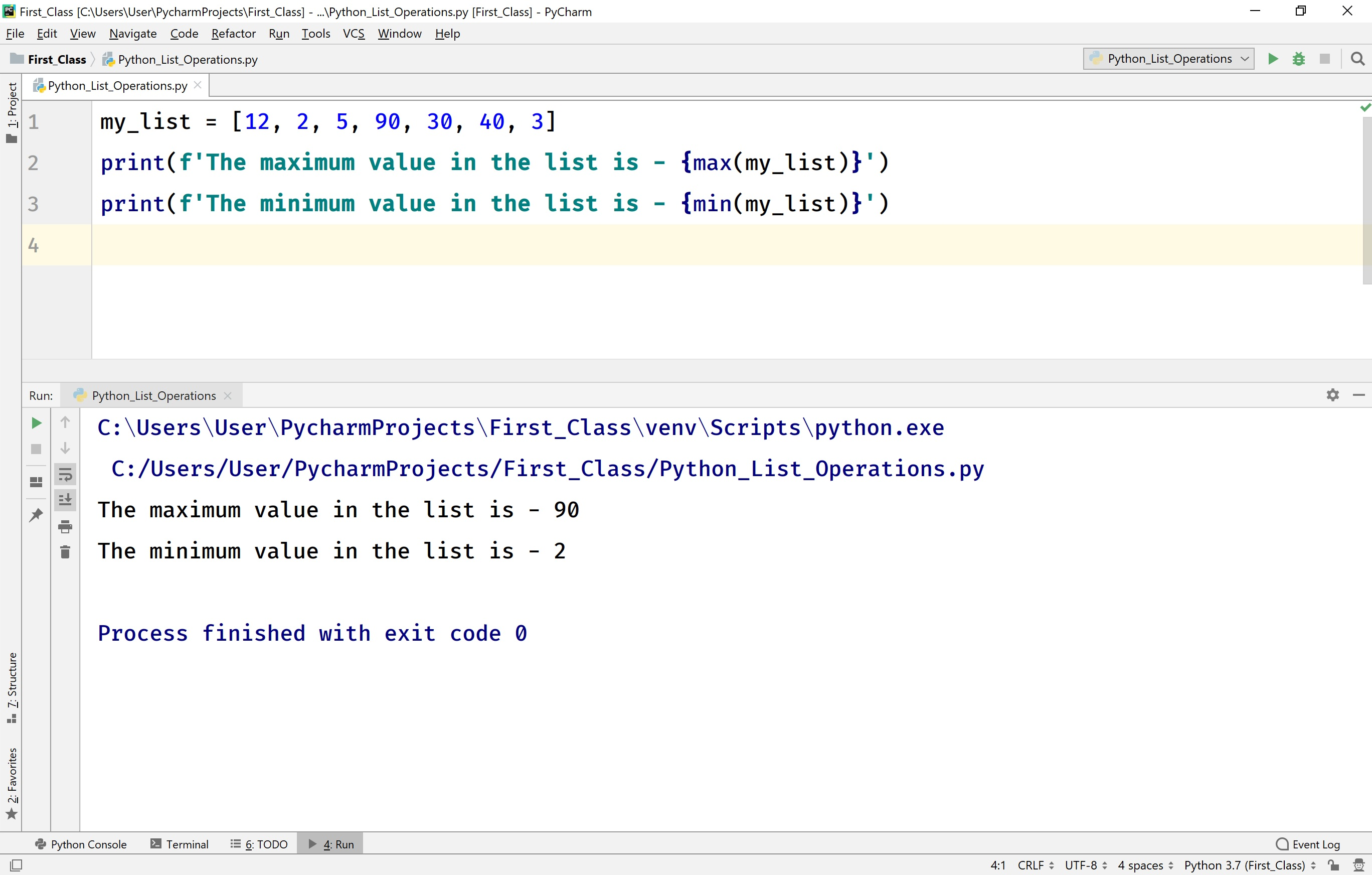## The reverse of a list

The Python provides a built-in method reverse( ) to produce the reverse of a list. The method reverse( ) returns None.

For example, consider the following code.

# Python code to illustrate reverse( ) in a list.

``````
my_list = [12, 2, 5, 90, 30, 40, 3]
print(f'The list is - {my_list}')
my_list.reverse()
print(f'Reverse of the list is - {my_list}')
```
```

When we run the above code, it produces the output as follows.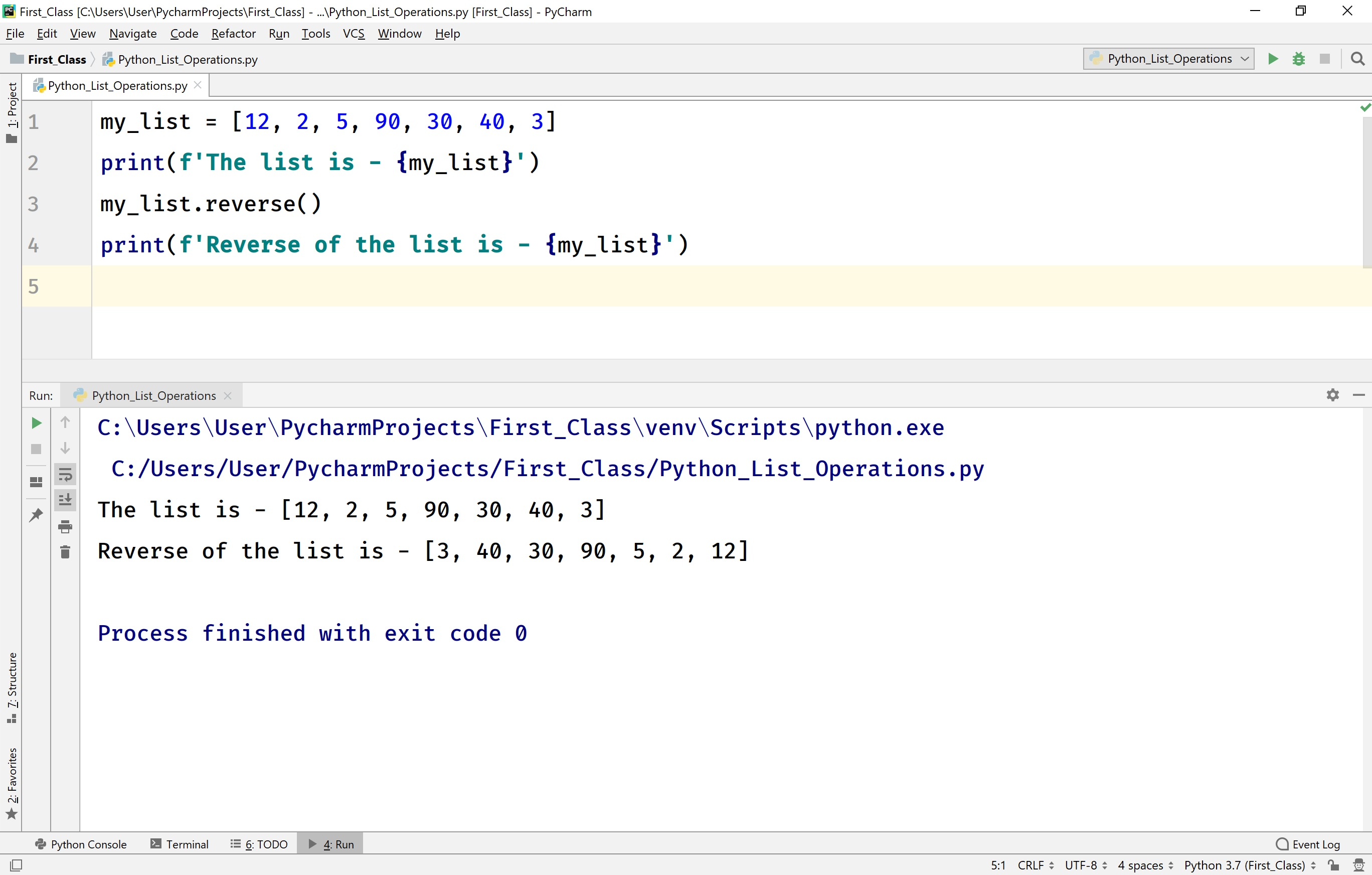## Sorting the elements of a list

The Python provides a built-in method sort( ) to sort all the elements of a list. The method sort( ) returns None. The sort( ) method arranges the list elements in increasing order by default. To sort the elements in decreasing order, we need to pass an argument reverse = True to sort( ) method.

For example, consider the following code.

# Python code to illustrate sort( ) in a list.

``````
my_list = [12, 2, 5, 90, 30, 40, 3]
print(f'The list is - {my_list}')
my_list.sort()
print(f'Increasing order of the list is - {my_list}')
my_list.sort(reverse=True)
print(f'Decreasing order of the list is - {my_list}')
```
```

When we run the above code, it produces the output as follows.• In Python, the functions max( ), min( ), and sorted( ) can be used with the list only if the list contains all elements of numerical type.